ConicBundle
implemention of a PSCOracle (PSCAffineFunction)

PSCAffineFunction is an implementation of ConicBundle::PSCOracle for the minimization of the maximum eigenvalue of an affine matrix function or, equivalently, Lagrangian relaxation of Linear Programs over the cone of positive semidefinite matrices. More...

## Classes

class  ConicBundle::PSCAffineMinorantExtender
Implementation of MinorantExtender for PSCAffineFunction. More...

class  ConicBundle::PSCAffineFunction
general purpose implementation of PSCOracle as explained in implemention of a PSCOracle (PSCAffineFunction) More...

class  ConicBundle::PSCPrimal
PSCPrimal is the corresponding positive semidefinite object for PSCOracle like PrimalMatrix for a MatrixFunctionOracle. More...

class  ConicBundle::PSCAffineModification
class for collecting and organizing a sequence of changes to block diagonal symmetric affine matrix functions so that it can be carried out in one step later on; More...

class  ConicBundle::CoeffmatInfo
allows to memorize the scalings applied to a Coeffmat and offers the basis for storing further user defined informations on a Coeffmat More...

class  ConicBundle::CMIName
extends CoeffmatInfo to store a name (e.g. of the constraint it represents) More...

class  ConicBundle::Coeffmat
defines a base class for coefficient matrices in semidefinite programming, in particular for use with MatrixSDPfunction, see implemention of a PSCOracle (PSCAffineFunction). More...

class  ConicBundle::CoeffmatPointer
pointer class for Coeffmat for deleting objects on the heap if Coefmat::use_cnt is reduced to zero and deletion is allowed. More...

class  ConicBundle::CMsymdense
implements a general dense symmetric Coeffmat based on CH_Matrix_Classes::Symmatrix (for use with MatrixSDPfunction, see implemention of a PSCOracle (PSCAffineFunction)). More...

class  ConicBundle::CMsymsparse
implements a general sparse symmetric Coeffmat based on CH_Matrix_Classes::Sparsesym (for use with MatrixSDPfunction, see implemention of a PSCOracle (PSCAffineFunction)). More...

class  ConicBundle::CMgramdense
implements a Gram matrix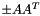as Coeffmat for a dense rectangular CH_Matrix_Classes::Matrix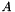(for use with MatrixSDPfunction, see implemention of a PSCOracle (PSCAffineFunction)). More...

class  ConicBundle::CMgramsparse
implements a Gram matrixas Coeffmat for a sparse rectangular CH_Matrix_Classes::Sparsemat(for use with MatrixSDPfunction, see implemention of a PSCOracle (PSCAffineFunction)). More...

class  ConicBundle::CMgramsparse_withoutdiag
implements a Gram matrix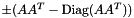with zero diagonal as Coeffmat for a sparse rectangular CH_Matrix_Classes::Sparsemat(for use with MatrixSDPfunction, see implemention of a PSCOracle (PSCAffineFunction)). More...

class  ConicBundle::CMlowrankdd
implements a low rank matrix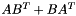as Coeffmat with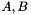each a dense rectangular CH_Matrix_Classes::Matrix (for use with MatrixSDPfunction, see implemention of a PSCOracle (PSCAffineFunction)). More...

class  ConicBundle::CMlowranksd
implements a low rank matrixas Coeffmat witha sparse rectangular CH_Matrix_Classes::Sparsemat and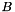a dense rectangular CH_Matrix_Classes::Matrix (for use with MatrixSDPfunction, see implemention of a PSCOracle (PSCAffineFunction)). More...

class  ConicBundle::CMlowrankss
implements a low rank matrixas Coeffmat witheach a sparse rectangular CH_Matrix_Classes::Sparsemat (for use with MatrixSDPfunction, see implemention of a PSCOracle (PSCAffineFunction)). More...

class  ConicBundle::CMsingleton
implements a Coeffmat having just one nonzero element (or two by symmetry) for use with MatrixSDPfunction, see implemention of a PSCOracle (PSCAffineFunction). More...

class  ConicBundle::Bigmatrix
AffineMatrixFunction needs to compute the maximum eigenvalue of an affine matrix function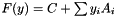. This class prepares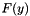in useful form for iterative eigenvalue solvers. More...

## Functions

ConicBundle::PSCAffineMinorantExtender::PSCAffineMinorantExtender (PSCAffineFunction *amf)
the PSCAffineFunction pointed to has to be valid for the livetime of this object

int ConicBundle::PSCAffineMinorantExtender::extend (Minorant &minorant, int n_coords, const int *indices)
called by ConicBundle to update internal Minorant objects, has to return 0 on success More...

int ConicBundle::PSCAffineFunction::form_bigmatrix (const CH_Matrix_Classes::Matrix &current_point)
compute the Bigmatrix representation for the given point

int ConicBundle::PSCAffineFunction::apply_modification (const PSCAffineModification &amfmod)
applies the PSCAffineModfication amfmod to the current function

void ConicBundle::PSCAffineFunction::clear ()
resets all to the initial empty state

## Variables

PSCAffineFunctionConicBundle::PSCAffineMinorantExtender::amf
the oracle this MinorantExtender was generated by, needed for retrieving problem data

SparseCoeffmatMatrix ConicBundle::PSCAffineFunction::C
block diagonal representation of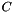as in implemention of a PSCOracle (PSCAffineFunction)

SparseCoeffmatMatrix ConicBundle::PSCAffineFunction::opAt
holds the coefficient matrices for the variables

PSCPrimalConicBundle::PSCAffineFunction::generating_primal
This PSCPrimal can be set from outside and serves for generating further primals by cloning.

std::vector< AMFMaxEigSolver * > ConicBundle::PSCAffineFunction::maxeigsolver
the eigenvalue solver of each block

CH_Matrix_Classes::Matrix ConicBundle::PSCAffineFunction::last_bigmat_y
the last y used in costructing the matrix of the affine matrix function

CH_Matrix_Classes::Integer ConicBundle::PSCAffineFunction::maxvecs
the maximum number of Ritz vectors to be retrurned

bool ConicBundle::PSCAffineFunction::check_correctness_flag
if true, ConicBundle employs some additional consistency checks

## Initialization and setting parameters

ConicBundle::PSCAffineFunction::PSCAffineFunction (const CBout *cb=0, int incr=-1)

ConicBundle::PSCAffineFunction::PSCAffineFunction (const SparseCoeffmatMatrix &C, const SparseCoeffmatMatrix &opAt, PSCPrimal *generating_primal=0, const CBout *cb=0, int incr=-1)
initialize the PSCAffineFunction with its matrices and possible a generating_primal More...

ConicBundle::PSCAffineFunction::~PSCAffineFunction ()

void ConicBundle::PSCAffineFunction::set_check_correctness (bool chk)
set the maximum number of Ritz vectors returned in evaluate(), see implemention of a PSCOracle (PSCAffineFunction) More...

void ConicBundle::PSCAffineFunction::set_max_Ritzvecs (CH_Matrix_Classes::Integer maxv)
set the maximum number of new Ritzvectors returned by evaluate(); values<1 default to 5

## Implementations of PSCOracle routines

MinorantConicBundle::PSCAffineFunction::generate_minorant (const CH_Matrix_Classes::Matrix &P)
see PSCOracle::generate_minorant()

int ConicBundle::PSCAffineFunction::svec_projection (CH_Matrix_Classes::Matrix &svec_offset, CH_Matrix_Classes::Matrix &svec_coeffs, const CH_Matrix_Classes::Matrix &P, const CH_Matrix_Classes::Indexmatrix *index_subset=0)
see PSCOracle::svec_projection()

int ConicBundle::PSCAffineFunction::evaluate (const CH_Matrix_Classes::Matrix &current_point, const CH_Matrix_Classes::Matrix &bundlevecs, const double relprec, const double Ritz_bound, CH_Matrix_Classes::Matrix &Ritz_vectors, CH_Matrix_Classes::Matrix &Ritz_values, PSCPrimalExtender *&primal_extender)
see PSCOracle::evaluate()

int ConicBundle::PSCAffineFunction::evaluate_projection (const CH_Matrix_Classes::Matrix &current_point, const CH_Matrix_Classes::Matrix &P, const double relprec, CH_Matrix_Classes::Matrix &projected_Ritz_vectors, CH_Matrix_Classes::Matrix &projected_Ritz_values)
see PSCOracle::evaluate_projection()

int ConicBundle::PSCAffineFunction::left_right_product (int i, const CH_Matrix_Classes::Matrix &E, const CH_Matrix_Classes::Matrix &F, CH_Matrix_Classes::Matrix &G)
see PSCOracle::left_right_product()

virtual int ConicBundle::PSCAffineFunction::apply_modification (const OracleModification &oracle_modification, const CH_Matrix_Classes::Matrix *new_center, const CH_Matrix_Classes::Matrix *old_center, bool &discard_objective_in_center, bool &discard_model, bool &discard_aggregates, MinorantExtender *&minorant_extender)
see PSCOracle::apply_modification() for the general use, here oracle_modification has a special role if it can be cast to an PSCAffineModification More...

virtual bool ConicBundle::PSCAffineFunction::check_correctness () const
see PSCOracle::check_correctness()

## routines for querying data of the problem

const SparseCoeffmatMatrixConicBundle::PSCAffineFunction::get_opAt ()

const SparseCoeffmatMatrixConicBundle::PSCAffineFunction::get_C ()

const PSCPrimalConicBundle::PSCAffineFunction::get_generating_primal (void)
returns the current setting concerning the generation of an PSCPrimal (0 for none)

## routines for supporting input and output

void ConicBundle::PSCAffineFunction::set_out (std::ostream *o=0, int pril=1)
see ConicBundle::CBout

void ConicBundle::PSCAffineFunction::set_cbout (const CBout *cb, int incr=-1)
see ConicBundle::CBout

std::ostream & ConicBundle::PSCAffineFunction::print_problem_data (std::ostream &out) const
write the problem description to out so that it can be read again by read_problem_data()

clear() and read the problem from in in the format written by print_problem_data()

std::ostream & ConicBundle::PSCAffineFunction::print_problem_data_to_mfile (std::ostream &out, CH_Matrix_Classes::Integer blocknr) const
undocumented highly volatile variant for external testing

typedef std::vector< CoeffmatPointerConicBundle::CoeffmatVector
convenient for initializing SparseCoeffmatMatrix via the sparse (block_i,column_i,Coeffmat_i), i=1,...,nz (nonzeros) format with Indexmatrix,Indexmatrix,CoeffmatVector

typedef std::map< CH_Matrix_Classes::Integer, CoeffmatPointerConicBundle::SparseCoeffmatVector
this is used to extract a row/block or a column from a SparseCoeffmatMatrix

enum  ConicBundle::Coeffmattype {
ConicBundle::CM_unspec =0, ConicBundle::CM_symdense =1, ConicBundle::CM_symsparse =2, ConicBundle::CM_lowrankdd =3,
ConicBundle::CM_lowranksd =4, ConicBundle::CM_lowrankss =5, ConicBundle::CM_gramdense =6, ConicBundle::CM_gramsparse =7,
ConicBundle::CM_singleton =8, ConicBundle::CM_gramsparsewd =9
}
for recognizing the type when writing and reading the problem More...

CoeffmatInfoConicBundle::clone (const CoeffmatInfo *cip)
if cip is not zero, it calls and returns cip->clone() and 0 otherwise

reads the next Coeffmat from in into an object on the heap and returns a pointer to it. The caller has to destruct the object.

## Detailed Description

PSCAffineFunction is an implementation of ConicBundle::PSCOracle for the minimization of the maximum eigenvalue of an affine matrix function or, equivalently, Lagrangian relaxation of Linear Programs over the cone of positive semidefinite matrices.

The class PSCAffineFunction implements a general purpose version of PSCOracle for minimizing the (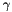-weighted) maximum eigenvalue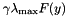of an affine matrix function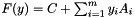, see abstract positive semidefinite cone oracle for an explanation of the general setting and the connections to Lagrangian relaxation of SDPs of the form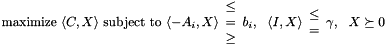PSCAffineFunction supports a rich variety of special choices for the matricesand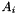and uses (for large matrices) an iterative Lanczos method to compute eigenvalues and eigenvectors of. In particular, the matricesandmay consist of a single or several diagonal blocks (the dimensions of the blocks have to be consistent forand the), each block being a coefficient matrix of the abstract class ConicBundle::Coeffmat . The following versions of this abstract class are implemented so far and may be used directly as blocks

The block diagonal coefficient matricesandare each organized as instances of a ConicBundle::SparseCoeffmatMatrix which has the blocks as rows and each of its columns corresponds to one symmetric block matrix consisting of the corresponding diagonal blocks. In fact, it is more useful to think of the diagonal blocks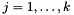as corresponding to separate semidefinite variables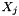, so that for each block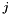there are coefficient matrices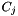and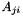,(many possibly of value zero)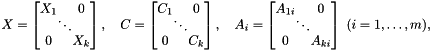resulting in an SDP problem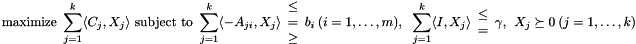In this sense ConicBundle::SparseCoeffmatMatrix is simply a representation of a sparse matrix having a (pointer to a) matrixas element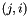. In PSCAffineFunction this matrix is called opAt.

The standard use of an PSCAffineFunction is to fully initialize it on construction with matrices C and opAt of type SparseCoeffmatMatrix (and maybe a generating primal, see below) and then to add this function to the MatrixCBSolver by MatrixCBSolver::add_function(). If dynamic changes to this PSCAffineFunction are required afterwards, use the class ConicBundle::PSCAffineModification within the corresponding problem modification routines MatrixCBSolver::append_variables(), MatrixCBSolver::reassign_variables(), MatrixCBSolver::delete_variables() of the MatrixCBSolver interface.

In order to allow the solver (or rather PSCModel) to aggregate the eigenvector information to primal approximations of the primal semidefinite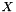(or the semidefinite blocks), one may install a pointer to a generating PSCPrimal on construction or via applying PSCAffineModification::add_reset_generating_primal(). Several versions of PSCPrimal are implemented and ready to use:

• DensePSCPrimal, general purpose densebased on CH_Matrix_Classes::Symmatrix, this is only useful for small matrices or small blocks, but it also keeps all information.
• SparsePSCPrimal, it is initialized with a nonzero support structure and then the matrix entries are only collected on this support. The implementation is based on CH_Matrix_Classes::Sparsesym and is particularly useful if all matricesandhave a common small support, because then all inner products are formed correctly with this reduced support of the true.
• GramSparsePSCPrimal, this is tuned for best representing the current optimal solution of the bundle subproblem of the solver (or rather PSCModel) by a matrix of the form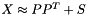where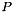is the part due to the subspace description of the model and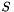is the sparse part collected for the aggregate matrices of the model on a selected support as in SparsePSCPrimal.
• BlockPSCPrimal allows to represent the primal information of a diagonal block partition of the fullby any choice of an PSCPrimal, in particular the ones given before. ifis itself a block matrix, typically the block partition of BlockPSCPrimal will be chosen to match the one ofbut it is not required to match. One main purpose in this choice is to allow inner products to work correctly for any new contraintsthat are added dynamically.

When the solver (or rather PSCModel) calls the implementation of PSCOracle::evaluate() for some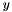, PSCAffineFunction collects the affine matrix function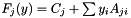of each blockin a separate Bigmatrix, the latter is derived from a CH_Matrix_Classes::Lanczosmatrix so as to serve as input for the iterative Lanczos eigenvalue solver CH_Matrix_Classes::Lanczpol. Then PSCAffineFunction starts a separate eigensolver (but so far not in parallel) for the bigmatrix of each block. If the block is small, a standard eigenvalue method is used, otherwise the Lanczos method is employed. In any case the method will not only yield the maximum eigenvector (or a Ritz vector with sufficiently large Ritz value to ensure a null step), but several other Ritz vectors as well, that might help to improve the quality of the model in PSCModel. The routine PSCAffineFunction::set_max_Ritzvecs() allows to specify how many of the Ritz vectors should be passed on to PSCModel as a result of the call to PSCAffineFunction::evaluate().

For facilitating input and output PSCAffineFunction offers

## ◆ Coeffmattype

for recognizing the type when writing and reading the problem

Enumerator
CM_unspec

any user defined constraint may use this

CM_symdense

for CMsymdense

CM_symsparse

for CMsymsparse

CM_lowrankdd

for CMlowrankdd

CM_lowranksd

for CMlowranksd

CM_lowrankss

for CMlowrankss

CM_gramdense

for CMgramdense

CM_gramsparse

for CMgramsparse

CM_singleton

for CMsingleton

CM_gramsparsewd

## ◆ apply_modification()

 virtual int ConicBundle::PSCAffineFunction::apply_modification ( const OracleModification & oracle_modification, const CH_Matrix_Classes::Matrix * new_center, const CH_Matrix_Classes::Matrix * old_center, bool & discard_objective_in_center, bool & discard_model, bool & discard_aggregates, MinorantExtender *& minorant_extender )
virtual

see PSCOracle::apply_modification() for the general use, here oracle_modification has a special role if it can be cast to an PSCAffineModification

if oracle_modification cannot be cast to an PSCAffineModification it is assumed that all append modifications amount to have already been carried out on *this seperately before this routine is called. In particular, it is only checked whether the new dimension matches the one given by oracle_modification, the old dimension is ignored. If this does not hold, the routine stops with an error. Otherwise it checks the other stuff as if a suitable PSCAffineModification has just been executed.

Reimplemented from ConicBundle::PSCOracle.

## ◆ extend()

 int ConicBundle::PSCAffineMinorantExtender::extend ( Minorant & minorant, int n_coords, const int * indices )
virtual

called by ConicBundle to update internal Minorant objects, has to return 0 on success

Parameters
 [in,out] minorant (Minorant&) it holds a (possibly aggregated) minorant that was generated from minorants returned by oracle calls, e.g. as in FunctionOracle::evaluate() If PrimalData was provided in these minorants, this will be aggregated along and will also be available in this minorant. [in] n_coords (int) the number of coordinate positions that have to be filled in [out] indices (const int*) the indices of these coordinate positions (sorted in strictly increasing order)
Returns
• 0 on success,
• 1 if extension is impossible

Implements ConicBundle::MinorantExtender.

## ◆ get_C()

 const SparseCoeffmatMatrix& ConicBundle::PSCAffineFunction::get_C ( )
inline

returns the block representation of the coefficient matrices (each entry of the map represents a block by a SparseCoeffmatVector).

Referenced by ConicBundle::PSCModel::QPPSCOracleData::get_C().

## ◆ get_opAt()

 const SparseCoeffmatMatrix& ConicBundle::PSCAffineFunction::get_opAt ( )
inline

returns the row representation of the coefficient matrices (each entry of the map represents a row by a SparseCoeffmatVector).

Referenced by ConicBundle::PSCModel::QPPSCOracleData::get_opAt().

## ◆ PSCAffineFunction()

 ConicBundle::PSCAffineFunction::PSCAffineFunction ( const SparseCoeffmatMatrix & C, const SparseCoeffmatMatrix & opAt, PSCPrimal * generating_primal = 0, const CBout * cb = 0, int incr = -1 )

initialize the PSCAffineFunction with its matrices and possible a generating_primal

C and opAt define the constant (block-)offset and the linear (block-)matrix function as described in the general text of PSCAffineFunction

generating_primal defines in what form primal matrices should be aggregated. If the argument is NULL then no primal aggregation will take place. The control over the generating primal is passed over to this. This will delete an existing generating primal whenever a new generating primal is set or upon destruction.

The final two arguments allow to set the output, see CBout.

## ◆ set_check_correctness()

 void ConicBundle::PSCAffineFunction::set_check_correctness ( bool chk )
inline

set the maximum number of Ritz vectors returned in evaluate(), see implemention of a PSCOracle (PSCAffineFunction)

if set to true, ConicBundle employs some additional consistency checks